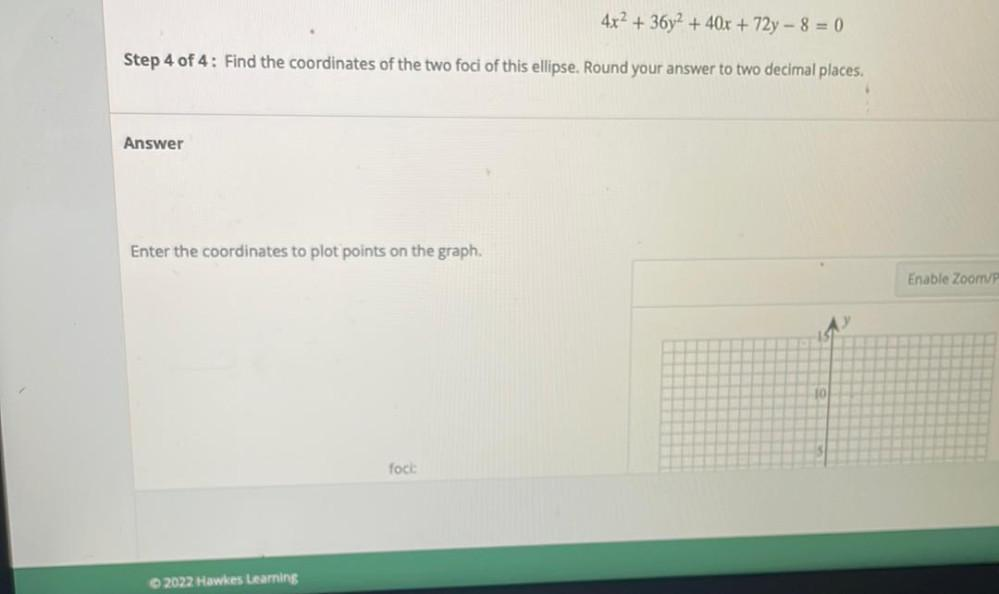Question:

# 4x2 + 36y2 + 40x + 72y - 8 = 0 Step 4 of 4: Find the coordinates of the two foci of this ellipse. Round your answer to two decim4x2 + 36y2 + 40x + 72y - 8 = 0 Step 4 of 4: Find the coordinates of the two foci of this ellipse. Round your answer to two decimal places. Answer Enter the coordinates to plot points on the graph. Enable Zoom fock: © 2022 Hawkes Learning